﻿ Vibration of Single-Walled Carbon Nanotubes by Using Nonlocal Theory

### Vibration of Single-Walled Carbon Nanotubes by Using Nonlocal Theory

Jozef Bocko, Pavol LengvarskýOPEN ACCESSPEER-REVIEWED

## Vibration of Single-Walled Carbon Nanotubes by Using Nonlocal Theory

Jozef Bocko1,, Pavol Lengvarský1

1Department of Applied Mechanics and Mechanical Engineering, Technical University of Košice, Faculty of Mechanical Engineering, Košice, Slovakia

### Abstract

The free bending vibration of single-walled carbon nanotubes (SWCNTs) is investigated in the present paper. A continuum approach based on nonlocal theory of beam bending is used for natural frequency computation. Analytical solutions of frequency equations are given for four types boundary conditions: clamped-free (C-F), simply-simply supported (S-S), clamped-simply supported (C-S) and clamped-clamped (C-C). The graphical representations of numerical results are shown for the first case of boundary conditions clamped end - free end.

### At a glance: Figures

1234
Prev Next

• Bocko, Jozef, and Pavol Lengvarský. "Vibration of Single-Walled Carbon Nanotubes by Using Nonlocal Theory." American Journal of Mechanical Engineering 2.7 (2014): 195-198.
• Bocko, J. , & Lengvarský, P. (2014). Vibration of Single-Walled Carbon Nanotubes by Using Nonlocal Theory. American Journal of Mechanical Engineering, 2(7), 195-198.
• Bocko, Jozef, and Pavol Lengvarský. "Vibration of Single-Walled Carbon Nanotubes by Using Nonlocal Theory." American Journal of Mechanical Engineering 2, no. 7 (2014): 195-198.

 Import into BibTeX Import into EndNote Import into RefMan Import into RefWorks

### 1. Introduction

Carbon nanotubes and underlying research are connected with Iijima and his famous paper . Carbon nanotubes are closed graphene sheets of cylindrical shape with interesting mechanical properties. Especially, high tensile strength and stiffness in relation to very small weight attracts attention of engineers and researches. However, not only mechanical properties of nanotubes are interesting. Intensive research is also oriented to the application in electrical and chemical engineering, as well as in biological sciences. There have been some researches on the vibration problems of SWCNTs through experiments, but at the same time theoretical treatment is used for description of measured data. Three basic methods of investigation are used to accomplish simulation of SWCNTs: molecular dynamic simulations, atomistic-based modeling and the continuum approach. The last one is used here for description of bending vibration and accordingly it can serve as a mean for indirect specification of carbon nanotube properties. The continuum beam relations used in the paper are based on the theory of nonlocal elasticity published by Eringen . In contrast to the classical elasticity theory Eringen takes into account the scale effect and its influence to stress at the reference point of a body. Accordingly, the stress at a point is a function of strain field at every point of body. Review of nonlocal elastic models of carbon nanotubes is given in  and nonlocal theory based on Euler beams is described in . The interesting results of SWCNT research can be found in literature [1-6,8,10,13,15].

### 2. Bending Vibrations of Carbon Nanotubes

2.1. Theoretical Background

The classical elasticity theory uses assumption of local action of physical quantities. It means that the stress at a given point correspondence to the strains in its intimate neighborhoods. In the nonlocal theory the stress state at the point of question is influenced by strains in all points of the investigated body . In accordance with this assumption, the behavior of homogeneous isotropic beam can be described by the following equation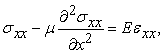(1)

where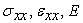are normal stress, normal strain and Young’s modulus, respectively. The scaling factor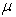at the second term on the left side of equation reflects the effect of small length scale. This factor can be expressed by equation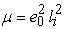, where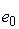is the material constant and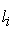is an internal characteristic length. In classical theory, parameteris used for modeling of bending, buckling and free vibration problems of beams . The Euler assumptions for free vibration of beam together with the nonlocal theory lead to equation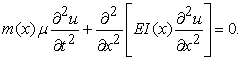(2)

Here,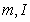and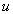are the mass per unit length, the moment of inertia of cross-section area and the transverse displacement of a nanotube, respectively. Fourier method of separation of variables leads to the system of two ordinary differential equations. One equation is related to time variable and the second one is connected with spatial variable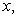describing axial position of point in question. Assumption that nanotube has a constant cross-section area leads to differential equation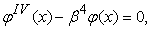(3)

where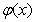is an eigenshape (mode shape) and for parameter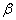we have equation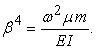(4)

The quantity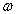in equation (4) represents an angular frequency of free vibration of nanotube and by usingthe equation (3) leads to the solution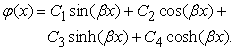(5)

Here,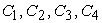are the constants depending on boundary conditions applied to the ends of investigated beam.

2.2. Solutions that Correspond to Different Boundary Conditions

Free vibrations of the armchair type of carbon nanotubes with boundary conditions: clamped-free (C-F), simply-simply supported (S-S), clamped-simply supported (C-S) and clamped-clamped (C-C) are solved in the paper. The length of carbon nanotube is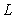and the lattice translation indices (n,m) for armchair nanotubes can be written as (n,n). Schema of graphene sheet and carbon nanotube is given in Figure 1.

The frequency equations for above-mentioned boundary conditions are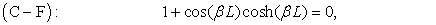(6)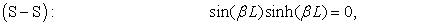(7)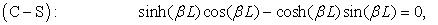(8)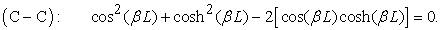(9)

The continuum approach leads to the fact that the frequency equations for given boundary conditions have infinite number of solutions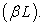The values of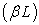for the first 15 eigenfrequencies (natural frequencies) were computed numerically in Matlab and they are given in Table 1.

The angular frequenciesare computed according to equation (4) and frequencies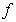from relation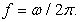In case of nanotubes there is uncertainty in definition of thickness of the nanotube shell. However, in the literature are given some relations that can be used in (4). Here, we apply relations from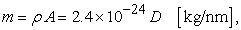(10)

#### Table 1. Solutions of Frequency EquationsDownload asPowerPoint Slide

Veiw figureView current table in a new window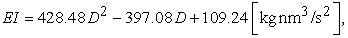(11)

where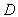is a diameter of nanotube. The nanotube diameter can be computed from translation indices (n,m) by relation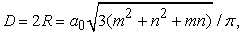(12)

where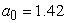Å is a carbon–carbon bond length and for armchair nanotube we have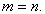Due to the fact that huge number of possible parameter combination can occur, the results are given only for the first four eigenfrequencies for diameters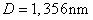and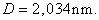The graphs on Figure 2 to Figure 11 show dependencies of eigenfrequencies on the length L of carbon nanotube with boundary conditions of type C-F. The nonlocal parameter is 0.01, 0.04, 0.1, 0.3 and 0.4, respectively.

Figure 11. The first four eigenfrequencies for diameter D=2,034 and nonlocal parameter μ=0.4

### 3. Conclusions

The paper deals with the free bending vibration of armchair single-walled carbon nanotubes. The study is based on the nonlocal beam theory, where the stress state at the investigated point is influenced by strains in all points of the investigated body. The solutions of frequency equations are given for four types of boundary conditions in the table and specific results for cantilever beam are given in several graphs. The eigenfrequencies are given there for two different diameters of nanotube, several nonlocal parameters and continuously changed length.

### Acknowledgement

This research was undertaken thanks to funding from Slovak Grant Agency VEGA 1/1205/12 and KEGA 054TUKE-4/2014.

### References

  Eringen, A.C., Nonlocal continuum field theories, Springer-Verlag, 2002.In article  Grupta, S.S., Barta, R.C., “Continuum structures equivalent in normal mode vibrations to single-waled carbon nanotubes”, Comp. Mater. Sci., 43, 715-723, 2008.In article CrossRef  Harik, V.M., “Mechanics of carbon nanotubes: applicability of the continuum-beam models”, Comp. Mater. Sci., 24, 328-342, 2002.In article CrossRef  Iijima, S., “Helical microtubules of graphitic carbon”, Nature, 345, 56-58, 1991.In article CrossRef  Li, C.Y., Chou, T., “A structural mechanics approach for the analysis of carbon nanotubes”, Int. J. of Solids and Struct., 40, 2487-2499, 2003.In article CrossRef  Lu, J., Chen, H., Lu, P., Zhang, P., “Research of natural frequency of single-walled carbon nanotube”, Chinese J. of Chem. Phys., 20, 525-530, 2007.In article CrossRef  Lu, P., Lee, H.P., Lu, C., Zhang, P.Q., “Application of nonlocal beam model for carbon nanotubes”, Int. J. of Solids and Struct., 44, 5289-5300, 2007.In article CrossRef  Muc, A., Banas, A., Chwal, M., “Free vibrations of carbon nanotubes with defects”, Mech. and Mechan. Eng., 17, 157-166, 2013.In article  Reddy, J.N., Pang, S.D., “Nonlocal continuum theories of beams for the analysis of carbon nanotubes”, J. of Appl. Phys., 103, 023511-1-023511-16, 2008.In article CrossRef  Sakhaee-Pour, A., Ahmadian, M.T., Vafai, A. “Vibrational analysis of single-walled carbon nanotubes using beam element”, Thin-walled struct., 47, 646-652, 2009.In article CrossRef  Swain, A., Roy, T., Nanda, B.K., “Vibration behaviour of single walled carbon nanotube using finite element”, Int. J. Theor. and Appl. Res. in Mech. Eng., 2, 129-133, 2013.In article  Thongyothee, C., Chucheepsakul, S., “Finite element modeling of van der Waals interaction for elastic stability of multi-walled carbon nanotubes”, Adv. Mater. Res., 55-57, 525-528, 2008.In article CrossRef  Thongyothee, C., Chucheepsakul, S., Li, T., “Nonlocal elasticity theory for free vibration of single-walled carbon nanotubes”, Adv. Mater. Res., 747, 257-260, 2013.In article CrossRef  Tserpes, K.I., Papanikos, P., “Finite element modeling of single-walled carbon nanotubes”, Comp. Part B: Eng., 36, 468-477, 2005.In article CrossRef  Wang, Q., Liew, K.M., “Application of nonlocal continuum mechanics to static analysis of micro-and nano-structures”, Physics Letters A, 363, 236-242, 2007.In article CrossRef